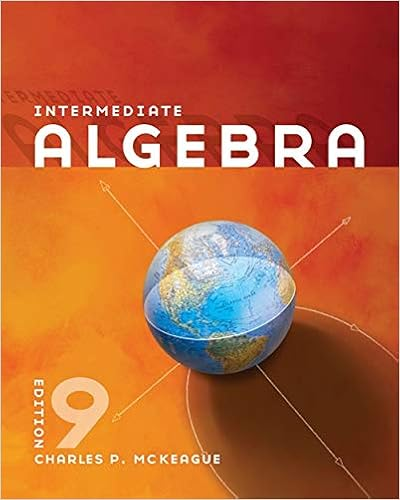# Week+2-Chapter+2+In+class+Activities+A+(1) (3).docx - Math...

• Homework Help
• 3
• 100% (1) 1 out of 1 people found this document helpful

This preview shows page 1 - 3 out of 3 pages.

##### We have textbook solutions for you!
The document you are viewing contains questions related to this textbook.The document you are viewing contains questions related to this textbook.
Chapter 11 / Exercise 6
Intermediate Algebra
MckeagueExpert Verified
Math 040: Re-Entry Mathematics Franklin UniversityWeek 2 Chapter 2 In class ActivitiesName: ____Rachelle Cuellar________________________ 1.Fill in the blank with < or > to make a true statementa.– 8> - 9 b.- 226 < 1982.Find the oppositea.31 ____-31_______b.-612 _____612______3.Simplify the expressiona.– (-15) __15_______b.|79|_____-79___c.– (34) _-34________d.|47|______47___4.Fill in the blank with <, >, or =a.– ( – 4) ___>__ |b.– 44 ____>___ – 54c.|55|_<_____ – ( – 65) for5.Add the numbers a.23 + 12 _______35___b.78 + (-33) __45_______c.-105 + ( -23) _-128_______d.-475 + (623) ___148_____6.Simplifya.20 + (−12) + (−5) __3____________b.−23 + (−4) + (−12) + (−5) ____-44________c.4 + (−12) + (−30) + 16 + 10 ___-12__________7.A contestant on Jeopardy scored the following amounts for several questions he answered. \$200, – \$400, \$1000, – \$400, \$600 Determine his total score.________600_________________________________ 8.Simplifya.35 (– 56) = __-21_________b.–320 – (–198) = ___-518________c.–40 + 605 – 815 = __-1460________d.–21 – 19 + (– 27) + 45 – (– 16) = ______-42________ 9.Temperatures on the Moon range from −184°C during its night to 214°C during its day. Find the difference between the highest temperature on the Moon and the lowest temperature. 4|_________398______________________________ 1030315
##### We have textbook solutions for you!
The document you are viewing contains questions related to this textbook.The document you are viewing contains questions related to this textbook.
Chapter 11 / Exercise 6
Intermediate Algebra
MckeagueExpert Verified
Math 040: Re-Entry Mathematics Franklin University
•••